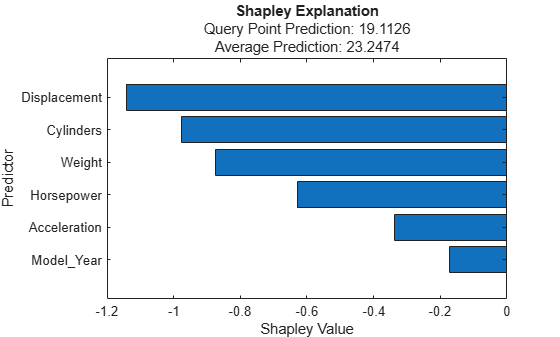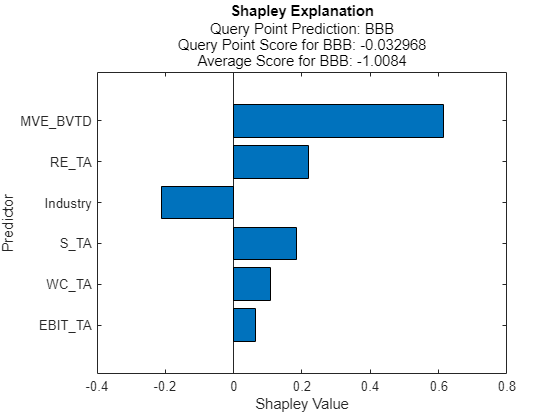# fit

Compute Shapley values for query point

## Syntax

``newExplainer = fit(explainer,queryPoint)``
``newExplainer = fit(explainer,queryPoint,Name,Value)``

## Description

example

````newExplainer = fit(explainer,queryPoint)` computes the Shapley values for the specified query point (`queryPoint`) and stores the computed Shapley values in the `ShapleyValues` property of `newExplainer`. The `shapley` object `explainer` contains a machine learning model and the options for computing Shapley values.`fit` uses the Shapley value computation options that you specify when you create `explainer`. You can change the options using the name-value arguments of the `fit` function. The function returns a `shapley` object `newExplainer` that contains the newly computed Shapley values.```

example

````newExplainer = fit(explainer,queryPoint,Name,Value)` specifies additional options using one or more name-value arguments. For example, specify `'UseParallel',true` to compute Shapley values in parallel.```

## Examples

collapse all

Train a regression model and create a `shapley` object. When you create a `shapley` object, if you do not specify a query point, then the software does not compute Shapley values. Use the object function `fit` to compute the Shapley values for the specified query point. Then create a bar graph of the Shapley values by using the object function `plot`.

Load the `carbig` data set, which contains measurements of cars made in the 1970s and early 1980s.

`load carbig`

Create a table containing the predictor variables `Acceleration`, `Cylinders`, and so on, as well as the response variable `MPG`.

`tbl = table(Acceleration,Cylinders,Displacement,Horsepower,Model_Year,Weight,MPG);`

Removing missing values in a training set can help reduce memory consumption and speed up training for the `fitrkernel` function. Remove missing values in `tbl`.

`tbl = rmmissing(tbl);`

Train a blackbox model of `MPG` by using the `fitrkernel` function

```rng('default') % For reproducibility mdl = fitrkernel(tbl,'MPG','CategoricalPredictors',[2 5]);```

Create a `shapley` object. Specify the data set `tbl`, because `mdl` does not contain training data.

`explainer = shapley(mdl,tbl)`
```explainer = shapley with properties: BlackboxModel: [1x1 RegressionKernel] QueryPoint: [] BlackboxFitted: [] ShapleyValues: [] NumSubsets: 64 X: [392x7 table] CategoricalPredictors: [2 5] Method: 'interventional-kernel' Intercept: 22.6202 ```

`explainer` stores the training data `tbl` in the `X` property.

Compute the Shapley values of all predictor variables for the first observation in `tbl`.

`queryPoint = tbl(1,:)`
```queryPoint=1×7 table Acceleration Cylinders Displacement Horsepower Model_Year Weight MPG ____________ _________ ____________ __________ __________ ______ ___ 12 8 307 130 70 3504 18 ```
`explainer = fit(explainer,queryPoint);`

For a regression model, `shapley` computes Shapley values using the predicted response, and stores them in the `ShapleyValues` property. Display the values in the `ShapleyValues` property.

`explainer.ShapleyValues`
```ans=6×2 table Predictor ShapleyValue ______________ ____________ "Acceleration" -0.1561 "Cylinders" -0.18306 "Displacement" -0.34203 "Horsepower" -0.27291 "Model_Year" -0.2926 "Weight" -0.32402 ```

Plot the Shapley values for the query point by using the `plot` function.

`plot(explainer)`The horizontal bar graph shows the Shapley values for all variables, sorted by their absolute values. Each Shapley value explains the deviation of the prediction for the query point from the average, due to the corresponding variable.

Train a classification model and create a `shapley` object. Then compute the Shapley values for multiple query points.

Load the `CreditRating_Historical` data set. The data set contains customer IDs and their financial ratios, industry labels, and credit ratings.

`tbl = readtable('CreditRating_Historical.dat');`

Train a blackbox model of credit ratings by using the `fitcecoc` function. Use the variables from the second through seventh columns in `tbl` as the predictor variables.

```blackbox = fitcecoc(tbl,'Rating', ... 'PredictorNames',tbl.Properties.VariableNames(2:7), ... 'CategoricalPredictors','Industry');```

Create a `shapley` object with the `blackbox` model. For faster computation, subsample 25% of the observations from `tbl` with stratification and use the samples to compute the Shapley values. Specify to use the extension to the kernelSHAP algorithm.

```rng('default') % For reproducibility c = cvpartition(tbl.Rating,'Holdout',0.25); tbl_s = tbl(test(c),:); explainer = shapley(blackbox,tbl_s,'Method','conditional-kernel');```

Find two query points whose true rating values are `AAA` and `B`, respectively.

```queryPoint(1,:) = tbl_s(find(strcmp(tbl_s.Rating,'AAA'),1),:); queryPoint(2,:) = tbl_s(find(strcmp(tbl_s.Rating,'B'),1),:)```
```queryPoint=2×8 table ID WC_TA RE_TA EBIT_TA MVE_BVTD S_TA Industry Rating _____ ______ ______ _______ ________ _____ ________ _______ 58258 0.511 0.869 0.106 8.538 0.732 2 {'AAA'} 82367 -0.078 -0.042 0.011 0.262 0.167 7 {'B' } ```

Compute and plot the Shapley values for the first query point.

```explainer1 = fit(explainer,queryPoint(1,:)); plot(explainer1)```Compute and plot the Shapley values for the second query point.

```explainer2 = fit(explainer,queryPoint(2,:)); plot(explainer2)```The true rating for the second query point is `B`, but the predicted rating is `BB`. The plot shows the Shapley values for the predicted rating.

`explainer1` and `explainer2` include the Shapley values for the first query point and second query point, respectively.

## Input Arguments

collapse all

Object explaining the blackbox model, specified as a `shapley` object.

Query point at which `fit` explains a prediction, specified as a row vector of numeric values or a single-row table.

• For a row vector of numeric values:

• The variables that makes up the columns of `queryPoint` must have the same order as the predictor data `X` in `explainer`.

• If the predictor data `explainer.X` is a table, then `queryPoint` can be a numeric vector if the table contains all numeric variables.

• For a single-row table:

• If the predictor data `explainer.X` is a table, then all predictor variables in `queryPoint` must have the same variable names and data types as those in `explainer.X`. However, the column order of `queryPoint` does not need to correspond to the column order of `explainer.X`.

• If the predictor data `explainer.X` is a numeric matrix, then the predictor names in `explainer.BlackboxModel.PredictorNames` and the corresponding predictor variable names in `queryPoint` must be the same. To specify predictor names during training, use the `'PredictorNames'` name-value argument. All predictor variables in `queryPoint` must be numeric vectors.

• `queryPoint` can contain additional variables (response variables, observation weights, and so on), but `fit` ignores them.

• `fit` does not support multicolumn variables or cell arrays other than cell arrays of character vectors.

If `queryPoint` contains `NaN`s for continuous predictors and `'Method'` is `'conditional-kernel'`, then the Shapley values (`ShapleyValues`) in the returned object are `NaN`s. Otherwise, `fit` handles `NaN` values in the same way as `explainer.BlackboxModel` (the `predict` object function of `explainer.BlackboxModel` or a function handle specified by `blackbox`).

Example: `explainer.X(1,:)` specifies the query point as the first observation of the predictor data `X` in `explainer`.

Data Types: `single` | `double` | `table`

### Name-Value Arguments

Specify optional pairs of arguments as `Name1=Value1,...,NameN=ValueN`, where `Name` is the argument name and `Value` is the corresponding value. Name-value arguments must appear after other arguments, but the order of the pairs does not matter.

Before R2021a, use commas to separate each name and value, and enclose `Name` in quotes.

Example: `fit(explainer,q,'Method','conditional-kernel','UseParallel',true)` computes the Shapley values for the query point `q` using the extension to the kernelSHAP algorithm, and executes the computation in parallel.

Maximum number of predictor subsets to use for Shapley value computation, specified as a positive integer.

For details on how `fit` chooses the subsets to use, see Computational Cost.

Example: `'MaxNumSubsets',100`

Data Types: `single` | `double`

Shapley value computation algorithm, specified as `'interventional-kernel'` or `'conditional-kernel'`.

• `'interventional-kernel'``fit` uses the kernelSHAP algorithm  with an interventional value function.

• `'conditional-kernel'``fit` uses the extension to the kernelSHAP algorithm  with a conditional value function.

For details about these algorithms, see Algorithms.

Example: `'Method','conditional-kernel'`

Data Types: `char` | `string`

Flag to run in parallel, specified as `true` or `false`. If you specify `'UseParallel',true`, the `fit` function executes for-loop iterations in parallel by using `parfor`. This option requires Parallel Computing Toolbox™.

Example: `'UseParallel',true`

Data Types: `logical`

## Output Arguments

collapse all

Object explaining the blackbox model, returned as a `shapley` object. The `ShapleyValues` property of the object contains the computed Shapley values.

To overwrite the input argument `explainer`, assign the output of `fit` to `explainer`:

`explainer = fit(explainer,queryPoint);`

collapse all

### Shapley Values

In game theory, the Shapley value of a player is the average marginal contribution of the player in a cooperative game. In the context of machine learning prediction, the Shapley value of a feature for a query point explains the contribution of the feature to a prediction (response for regression or score of each class for classification) at the specified query point.

The Shapley value of a feature for a query point is the contribution of the feature to the deviation from the average prediction. For a query point, the sum of the Shapley values for all features corresponds to the total deviation of the prediction from the average. That is, the sum of the average prediction and the Shapley values for all features corresponds to the prediction for the query point.

For more details, see Shapley Values for Machine Learning Model.

 Lundberg, Scott M., and S. Lee. "A Unified Approach to Interpreting Model Predictions." Advances in Neural Information Processing Systems 30 (2017): 4765–774.

 Aas, Kjersti, Martin. Jullum, and Anders Løland. "Explaining Individual Predictions When Features Are Dependent: More Accurate Approximations to Shapley Values." arXiv:1903.10464 (2019).